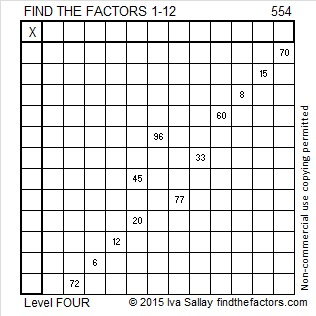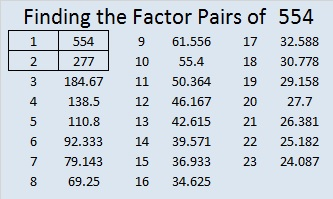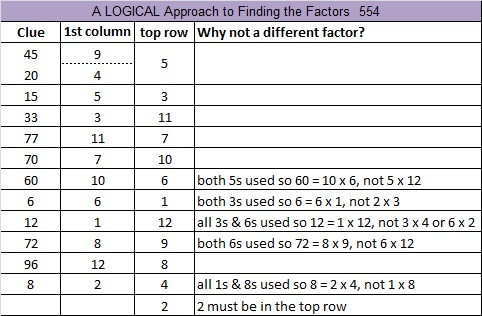# 554 and Level 4

554 is the hypotenuse of the Pythagorean triple 230-504-554. What is the greatest common factor of those three numbers?Print the puzzles or type the solution on this excel file: 12 Factors 2015-07-13

—————————————————————————————————

• 554 is a composite number.
• Prime factorization: 554 = 2 x 277
• The exponents in the prime factorization are 1 and 1. Adding one to each and multiplying we get (1 + 1)(1 + 1) = 2 x 2 = 4. Therefore 554 has exactly 4 factors.
• Factors of 554: 1, 2, 277, 554
• Factor pairs: 554 = 1 x 554 or 2 x 277
• 554 has no square factors that allow its square root to be simplified. √554 ≈ 23.5372—————————————————————————————————